Acoustic loss at substantial ultrasonic strain

in 6Al-6V-2Sn and

sintered 6Al-4V titanium

David Wuchinich

ã David Wuchinich 2004

Keywords:  Q, attenuation, dissipation, fatigue, titanium, ultrasonic, sintered, powdered metal, stress

PACS code:  74.75.Ld

# Abstract

The mechanical Q of the high strength 6Al-6V-2Sn titanium alloy and of two alloys of sintered 6Al-4V is measured and compared with values obtained for standard cast and rolled 6Al-4V at 20 kHz cyclic strains ranging for 0.18 to 0.42 percent.  6-6-2 was found to exhibit comparable loss to the standard samples while the sintered specimens revealed much lower dissipation.  Annealing of one of the sintered samples reduced the Q to those found typical for cast and rolled material.  The 6-6-2 material survived continuous operation at strains in the neighborhood of 0.4 percent.  It appears that sintering may provide an economical process for making ultrasonic horns and that intensities greater than can typically be produced by use of 6-4 Ti may be available using 6-6-2Ti.

### Introduction

Mechanical dissipation resulting from hysteresis in the stress-stain relationship in many metals has been studied extensively in relation to fatigue and the operational life of components subjected to cyclic stress at ultrasonic frequencies because this loss limits the maximum excursion that can reliably be produced.  As the alloy 6Al-4V titanium is used extensively in ultrasonic welding and surgical horns, and is often subjected to strains in the range of 0.25 percent during operation, Mason’s exemplary method and comprehensive studies have provided a guide for fatigue -safe design for this alloy.  A complete treatise of his methods has also been published.  Additional studies of similar alloys have produced comparable results:  The mechanical Q is constant for strains up to 0.25 percent, thereafter decreasing rapidly.  In this respect, this alloy of titanium is distinguished among other metals employed as horns, such as steel and aluminum, both of which exhibit progressively greater loss with increasing strain.  A strain of 0.25 percent corresponds to a stress of 276 Mpa (40 kpsi) and it is this value that has been adopted as the practical endurance limit for titanium horns operating at ultrasonic frequencies.

Horns and ultrasonic surgical tips have commonly been made by machining rolled stock taken from castings.  Recently, sintered versions of the alloy have become available and offer substantial economies in the manufacture of large quantities of identical items as the process results in horns having near to net finished shape from molds.  Sintering, however, may result in porosity which, if occurring in regions subject to substantial strain, can cause stress concentrations that precipitate failure.  Hence, an acoustic evaluation of this material’s tolerance of large strains is of interest.

Also of interest in this study is the performance of the titanium alloy 6Al-6V-2Sn, known for having higher strength than 6Al-4V and which has become available to users in common rolled bar form in less than mill run quantities.

## Theory

The half wavelength resonator utilized by Mason and which closely resembles in action the classical model of simple harmonic vibration utilizing two opposing and equal masses connected by a mass-less spring is a simple candidate for evaluation of acoustic loss.  A schematic representation of the structure is shown in Figure 1.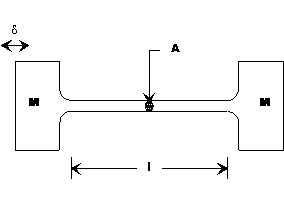Figure 1  Test specimen geometry

This model is chosen for two principal reasons:  (1) stress is confined to the spindle portion of the resonator and is substantially uniform in this region and (2) the abrupt transistion in cross sectional area between the mass and spring elements permit the production of large cyclic stress for modest and easily produced free end amplitude excursions.  As elastic loss is often a function of the peak stress level, confinement and uniformity of stress permits a reliable measurement of loss.  Production of stress levels likely to reveal substantial losses without resort to excitation by high intensity transducer and horn amplifiers is also an advantage.

Exact and finite element analysis reveal that indeed, for the first extensional resonant mode, the end sections with the larger diameter move uniformly in opposite directions and that the slender center section is subjected to substantially uniform strain with a frequency approximately given by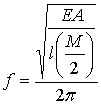(1)

with E representing Young’s modulus.

The stored energy of vibration, Es, can be computed as: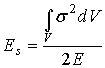(2)

where s is the peak stress and V the volume.  As the stress is principally located within the slender stem of length l whose cross section, A, is constant, Eq. (2) becomes: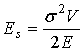.                                                     (3)

V = Al, the volume of the spindle.  The exact value can be computed from finite element modal analysis or from solutions for the wave equation for a model consisting of connected and appropriately sized prismatic steps , but Eq. (3) is sufficiently accurate for computations within fifteen percent of the true value if the geometry is chosen so that the stress is predominantly present in the stem.

The stress can be computed from optical measurements of the displacement of the end mass using, for example, a microscope with a calibrated reticule as: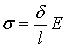(4)

where d is the excursion (peak to peak motion of either end mass) and l is the length of stem having a constant diameter.

Computation of the acoustic loss at any given level of vibration can be made by measurement of the temperature rise in the stem over a prescribed duration of vibration.  The rate of heat generation in the spindle can be written as: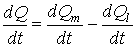(5)

where Q is the actual heat contained, Qm  is the heat generated by mechanical loss and Ql is the heat lost by conduction and radiation.

Measurement has shown that Ql can be accurately represented as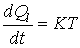(6)

where K is the heat loss coefficient and T is the difference between the spindle and ambient temperature.  K can be found by measuring the fall in temperature once vibration ceases (dQm/dt = 0).  Since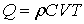with r the material density, C the specific heat capacity and V the spindle volume, it follows that: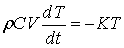from which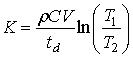(7)

where the temperature falls from T1 to T2  over an interval of time td.

Knowing K, equation 5 may be integrated and solved for Qm: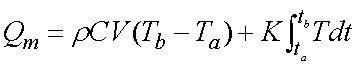(8)

Actual measurement has shown that the rise in temperature during vibration is closely proportional to the time so that T may be approximated as;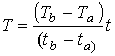where tbta is the interval of time over which the temperature rises from Ta to Tb .  Equation 8 can then be written as: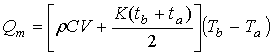(8a)

The energy dissipated per cycle of vibration, El, is then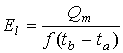(9)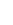where ¦ is the frequency of vibration

Q is defined as the ratio of the energy stored to that lost per cycle multiplied by 2p, and becomes, using the expressions for Es given by equation 3 and El  by equation 9 as: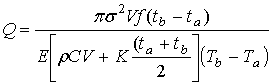(10)

### Method

Two samples of sintered 6Al-4V titanium were obtained in the form of rough cylindrical blanks.  Sample (#1) contained 10 percent titanium carbide.  The second sample (#2) had the conventional formulation of the cast material.  .  Figure 2 is a photograph of the samples as received.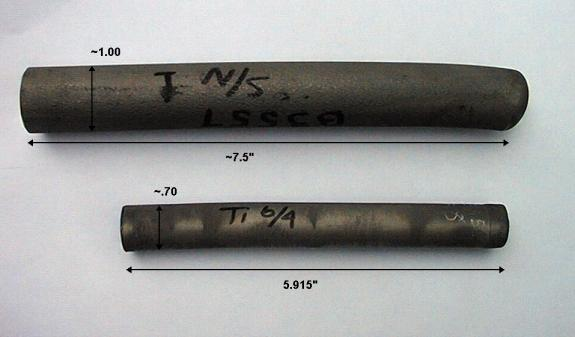Figure 2 - Sintered sample blanks as received

The extensional sound velocity, c, was found from the sample dimensions and by measuring the frequency of the first free-free flexural mode of vibration and the density obtained by measurement of displaced weight of water under suspended immersion.  Knowing both the density and sound velocity permitted computation of Young’s modulus: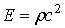(1)

where E is Young’s modulus, r the density and c the extensional sound velocity.  For both samples the density was found to be 4400 Kg/m3  (0.16 lbf/in3).  The sound velocity for sample 1 was computed from the flexural frequency to be 5100 m/s (200,000 in/s), while that for sample 2 was 5300 m/s (210,000 in/s).  Hence the moduli:

# Table 1

Density and Elastic moduli for sintered samples

 Sample Density, Kg/m3 (lbsf/in3) Modulus, GPa (Mpsi) 1 (10 percent TiC) 4400 (0.16) 117 (17) 2 (6-4) 4400 (0.16) 122 (18)

To determine the mechanical Q of these materials test specimens of Mason’s mass-spring-mass resonators, also known as spool or dumb bell resonators, were machined from each.  Figure 3 is a drawing of the powdered metal specimens designed to resonate at 20 kHz.Figure 3 – 20 kHz half wavelength acoustic test specimen

The specimens were attached to a 20 kHz transducer driven stepped horn as shown in Figure 4 using a cyanoacrylate adhesive.  The temperature rise in the center of the stem, which is a motional node, was made using a non-contacting infrared thermometer and confirmed by measurement using a Type K thermocouple.  The test duration varied with the level of applied vibration[DW1].

### Results

Table 2 provides a representative sample of the raw data.  The resonant frequency for all tests and both specimens was measured to be 19,990 Hz.  The temperature measurements shown are averages of at least three separate runs.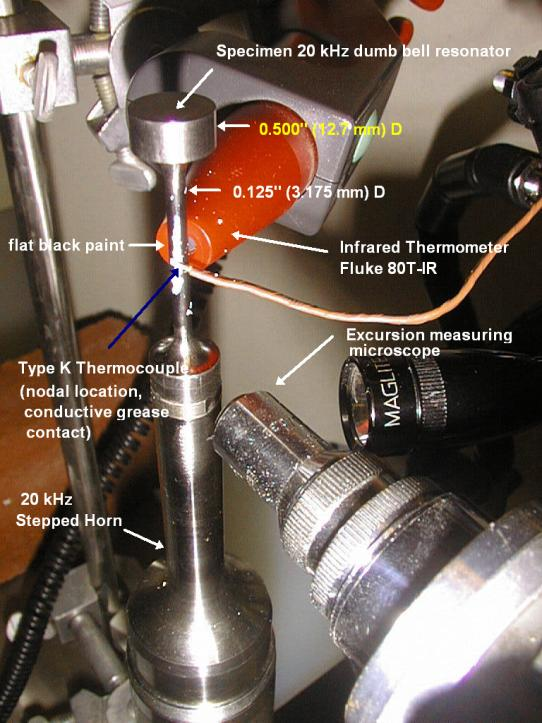Figure 4 - Testing apparatus arrangement

Table 2

20 kHz  Typical Thermal Measurement Data

 Specimen d, microns (.001in) Duration, s DTav ,oC (oF) Computed stress, MPa (kpsi) Strain % Comment 2 64 (2.5) 10 8.33 (15.0) 260 (37) 0.21 2 76 (3.0) 10 11.9 (21.5) 310 (44) 0.24

Figure 5 is a representative oscilloscope trace of the spindle temperature rise and fall during a single test.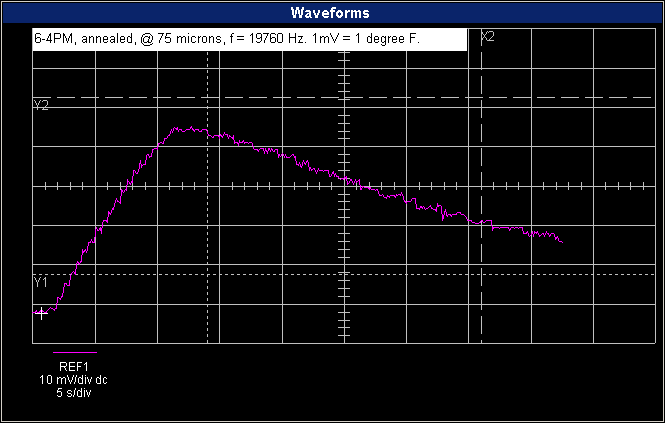Figure 5 - Spindle temperature variation with time

Figure 6 plots the Q, computed using the measured values of temperature rise and stress according to Eq. (6b), versus the strain.  The specific heat, C, of titanium was taken as 565 J/oK/kg (0.135 BTU/lb/oF = 1260 in.lbs/lb0F).  It is noted that Q’s computed without correction for heat loss were only ten percent larger than the values shown.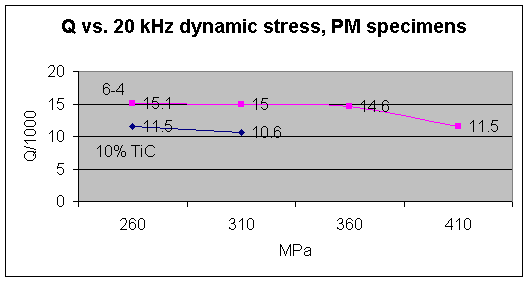Figure 6

The specimen containing 10 percent TiC fractured from repeated operation at 310 MPa cyclic stress.  The 6-4 specimen fractured when testing was attempted at 450 MPa.

Similar measurements were also made on a test specimen made of the alloy 6Al-6V-2Sn which was first annealed for one hour at 700 C (1300 F), followed by air cooling.  The pertinent material properties, again obtained by measurement of the flexural vibration frequency and density are tabulated below.

Table 3

Density and Elastic moduli for 6Al-6V-2Sn sample

 Density, Kg/m3 (lbsf/in3) Modulus, GPa (Mpsi) 4510 (0.163) 99.3 (14.4)

A plot of the Q of this sample versus operating cyclic stress is provided in Figure 7.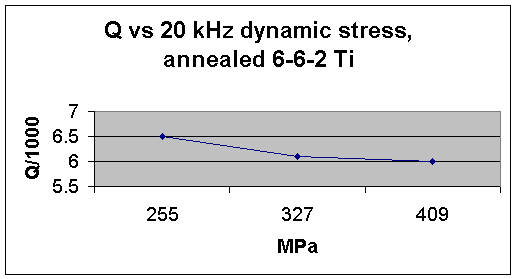Figure 7

Although this material exhibits a substantially lower Q than either of the sintered 6Al-4V samples, it was also tested for endurance at 414 Mpa (60 kpsi) operating cyclic stress with the spindle cooled by a water spray as shown in Figure 8.  The sample survived repeated 6 million-cycle operation at this stress level.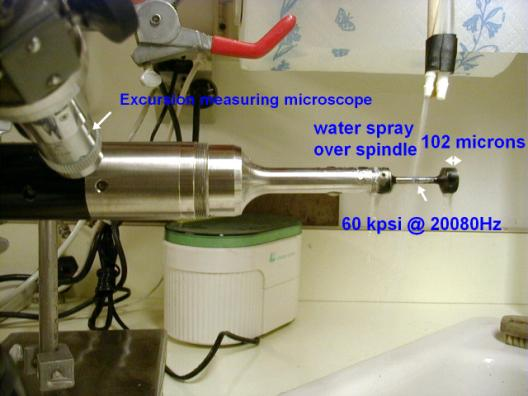Figure 8 – Endurance test of 6-6-2 Ti alloy

Test specimens were also made for two samples made from 6Al-4V round bar stock, revealing a Q of approximately 5000 at strains up to 0.33 percent.  Annealing of these samples did not affect the Q.

A sample of the 6-4 sintered material was also annealed at 730 C for one hour and allowed to air cool.  A specimen made from this sample was also tested.  Annealing reduced the Q from the values shown in Figure 6 to those found for standard rolled round stock.

As a check upon the thermal method applied here, samples of 6-6-2 and 6-4 specimens were cemented to an identically resonant transducer-booster combination whose quiescent power consumption at a measured vibration amplitude was known.  The additional power consumed at the same amplitude with the specimen attached is due to losses in the specimen and the Q can be computed by dividing the energy of vibration by the power lost per cycle.  The results obtained agreed with those listed here within ten percent.

Discussion

While the values of Q measured here are substantially below those found by Mason 3, all alloys evaluated appear comparable or superior in performance to the venerable 6Al-4V titanium alloy.

The Q of sintered 6Al-4V Ti appears to be substantially greater than that measured for cast and rolled material, and indicates that the material is a promising substitute for use in highly stressed ultrasonic horns as its acoustic losses begin to mount at the same stress threshold of approximately 275 Mpa (40 kpsi) exhibited by the conventional product.  The alloy containing 10 percent Titanium carbide and recommended for its ease in machining, while exhibiting more modest performance and progressive degradation at substantially lower dynamic stress, appears worthy of evaluation for use in applications where the maximum dynamic stress does not exceed 200 Mpa (30 kpsi).

The 6-6-2 alloy exhibits about the same losses as that shown in the samples made of common rolled 6-4 but it appears capable of sustaining cyclic strains in the neighborhood of 0.4 percent at 20 kHz in applications where cooling can be provided.

Acknowledgements

The author thanks Stanley Abkowitz of Dynamet Technology for discussion of sintered products’ properties and for supplying the samples and Professor Daniel Bershers of Columbia University for advice regarding his own and W.P. Mason’s dynamic strain studies.

 Modal Mechanics, 431 Hawthorne Avenue, Yonkers, NY 10705

 Neppiras, Mechanical transformers for producing very large motion, Letters to the editor, Acustica 13, 369-370, 1963.

Mason, W.P. and J. Wehr, Internal friction and ultrasonic yield stress of the alloy 90Ti6Al4V, J. Phys. Chem. Solids, 31:1925-1933, 1970.

` Mason, W.P., Low and high amplitude internal friction measurements in solids and their relation to imperfection motions, Advances in Material Research, Vol. 2, (C. J. McMahon, ed.), pp. 287-364, Interscience 1968.`

 Kuz’menko, V.A., Fatique strength of structural materials at sonic and ultrasonic loading frequencies, Ultrasonics 13:1, 21-30, 1975.

 Mason, W.P., Physical Acoustics and the Properties of Solids, p. 176, D. Van Nostrand, 1958

Puskar, A., Cyclic stress-strain curves and internal friction of steel at ultrasonic frequencies, Ultrasonics, May 1982.

 Dynamet Technology, Eight A Street, Burlington, MA 01803

 Merkulov, L.G. and A. V. Kharitonov, Theory and design of sectional concentrators, Soviet Physics – Acoustics, Vol. 5, 183-190, (1959).

 Roberts, M.H. and J. Nortcliffe, Measurement of Young’s Modulus at High Temperatures, J. Iron and Steel Inst., Nov. 1947, pp. 345-348.

 To avoid inadvertent stress concentration the reduction to the stem is radiussed, unlike that shown for Mason’s resonators.  See Methods of Experimental Physics, Vol. 19, Acoustics, p. 346, Academic Press, 1981.

 Computation using Eq.4 is within ten percent of these values obtained by both finite element modal analysis and boundary value solution of the wave equation applied to a step-wise model of the geometry.  Computation of Q using the simplified analysis is within fifteen percent of values tabulated.

[DW1] To view that formulae used in this note, open the note for editing.

To check the thermal measurements used to determine Q, the specimen was attached to a transducer/booster combination tuned to 20,000 Hz whose power dissipation at 3.0 mils excursion was measured (20 kHz xdcr&3to1 booster@3mils-*.wfd).  The average dissipation from ten independent measurements, obtained using power/t analysis in waveform manager, was found to be 5.14 watts with a standard deviation of 0.2 watts.  The specimen was then attached to the booster, using cyanoacrylate adhesive applied to lapped mating faces, and the power again measured ten times at the same excursion (45,000 psi peak cyclic stress).  The average power was found to be 7.5 watts with a standard deviation of 0.5 watts.

The average dissipation per cycle is then the difference between these averages divided by the frequency (20200 Hz when the specimen was attached, assuming that the dissipation of the booster/transducer combination does not change when the frequency shifts slightly from 20.000 to 20.2 kHz).

The energy equivalent mass, Me, which is the mass that if moved with the specimen's free end velocity produces the same kinetic energy as that stored in the specimen itself, was computed by adding a small mass, dm, to the specimen and computing, using FEA modal analysis, the new resonant frequency.  Me then can be found by evaluating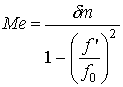, where f’' is the resonant frequency with mass dm added and fo is the frequency without the mass.  Knowing Me, the stored energy of vibration can be computed as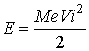where Vi is 2pf x, where f is the actual measured frequency of vibration and x is the optically measured excursion.

The mass added (SPOOL2') was 0.5 inches in diameter and 0.005 inches in length which, using a density of 0.163 lbsf/cu. in., gives 4.59E-5 slugs.  The frequency with the mass was measured to be 20010 Hz and with it, 19919 Hz.  Hence Me was found to be 4.59E-5 slugs and the stored energy of vibration computed to be 0.83 in lbs or 0.069 ft lbs or 0.093 J.

The Q is 2pE/dissipated energy per cycle.  As 2.35 additional watts were consumed with the specimen attached and the frequency measured was 20200 Hz, the energy dissipated per cycle is 1.16E-4 J, so that Q ~ 5,000.

Similar measurements were made of 6Al-4V at 3 mils excursion (44,000 psi peak cyclic stress) where the average power was found to be 6.81 watts with a standard deviation of 0.11 watts.  Using the frequency data from SPOOL and SPOOL' where the same mass was added to compute the energy equivalent mass, it is found that Me = 4.59E-5 slugs.  As the measurement was again made at a 3 mil excursion, E = 0.81 in lbs or .067 ft lbs = 0.091 J.  As the net dissipation is 6.81-5.14 = 1.67 watts or 8.43E-5 J/cycle (using a frequency of 19800 Hz), the Q is 6800.

Note:  Power measurements were made with the static capacitance of the transducer tuned with a series inductance of 10.1 mH, with the voltage measured between common and the inductor input and thus include any dissipation present in the inductor.  However, a measurement was also made directly across the transducer at 3 mils excursion.  The power measured, 6.95 watts average, was within the range of measured values.